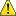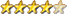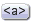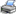Notice. New forum software under development. It's going to miss a few functions and look a bit ugly for a while, but I'm working on it full time now as the old forum was too unstable. Couple days, all good. If you notice any issues, please contact me.

Forum Index : Microcontroller and PC projects : Color Wheels

Author Message
William Leue
GuruJoined: 03/07/2020
Location: United States
Posts: 340
 Posted: 07:51pm 25 Aug 2020Copy link to clipboardPrint this post

Here is a short program that displays color wheels for the different color memory modes. There are 4 wheels which together cover most of the color gamut for each color memory mode.

It is interesting and instructive to see how the wheels change as you move from 8 to 12 and then 16-bit color. Whereas 8-bit color shows the expected color quantization and some false contouring, by the time you get to 16 bits the color shading is almost completely smooth and free from false contours.

No surprises there, and of course 8 bits is plenty for almost all games.

-Bill

' Color charts for the 3 color depths.
' Rev 1.0.0 William M Leue 8/21/2020

option default integer

const NMODES = 4
const HMARGIN = 50
const VMARGIN = 30
const CSEP = 30

do
ok = 1
input "Select Color Bits (8, 12, or 16): ", ans\$
b = val(ans\$)
if b <> 8 and b <> 12 and b <> 16 then
print "unsupported color bits - try again"
ok = 0
end if
loop until ok = 1

mode 1, b
cls
text HMARGIN+2*CRADIUS+CSEP/2, 280, str\$(b) + "-bit Colors", C

DrawColorWheels

xx\$ = INKEY\$
text 10, 585, "Press any Key to Exit"
do : loop until INKEY\$ <> ""
end

' Draw 4 color wheels for the current color bit mode:
' Top Left wheel: Brightness = 1, Saturation decreases toward center
' Top Right wheel: Saturation = 1, Brightness decreases toward center
' Bottom Left wheel: Brightness and Saturation both decrease.
' Bottom Right wheel: Grayscale.
sub DrawColorWheels
local cx, cy, x, y, sr
local integer r, g, b
local float hue, sat, brt, fr
local col

text cx, VMARGIN-2, "V=1.0, S=1.0->0.0", CB
for sr = CRADIUS-1 to 1 step -1
sat = sr/fr
for hue = 0.0 to 359.5 step 0.5
HSV2RGB hue, sat, 1.0, r, g, b
col = RGB(r, g, b)
box x, y, 2, 2,, col, col
next hue
next sr

text cx, VMARGIN-2, "S=1.0,V=1.0->0.0", CB
for sr = CRADIUS-1 to 1 step -1
brt = sr/fr
for hue = 0.0 to 359.5 step 0.5
HSV2RGB hue, 1.0, brt, r, g, b
col = RGB(r, g, b)
box x, y, 2, 2,, col, col
next hue
next sr

for sr = CRADIUS-1 to 1 step -1
sat = sr/fr
brt = sr/fr
for hue = 0.0 to 359.5 step 0.5
HSV2RGB hue, sat, brt, r, g, b
col = RGB(r, g, b)
box x, y, 2, 2,, col, col
next hue
next sr

for sr = CRADIUS-1 to 1 step -1
brt = sr/fr
for hue = 0.0 to 359.5 step 0.5
HSV2RGB hue, 0.0, brt, r, g, b
col = RGB(r, g, b)
box x, y, 2, 2,, col, col
next hue
next sr

end sub

sub PrintMsg msg\$
text 10, 550, SPACE\$(50)
text 10, 550, msg\$
end sub

sub GetInput msg\$, ans\$
PrintMsg msg\$
input ans\$
end sub

' Convert an RGB color value to its HSV equivalent
' the RGB values must be in range 0..255; the S and V values will
' be in range 0..1; the H value will be in range 0..360
sub RGB2HSV r, g, b, h, s, v
local rp, cmax, cmin, delta

rp = r/255.0 : gp = g/255.0 : bp = b/255.0
cmax = max(rp, max(gp, bp))
cmin = min(rp, min(gp, bp))
delta = cmax - cmin
if delta = 0 then
h = 0
else if cmax = rp then
h = 60*(((gp-bp)/delta) MOD 6)
else if cmax = gp then
h = 60*(((bp-rp)/delta) + 2)
else
h = 60*(((rp-gp)/delta) + 4)
end if
if cmax = 0 then
s = 0
else
s = delta/cmax
end if
v = cmax
end sub

' Convert an HSV value to its RGB equivalent
' The S and V values must be in range 0..1; the H value must
' be in range 0..360. The RGB values will be in range 0..255.
sub HSV2RGB h as float, s as float, v as float, r as integer, g as integer, b as integer
local float hh, f, p, q, t, x, c, rp, gp, bp
local integer i

c = v*s
hh = h/60.0
i = int(hh)
f = hh - i
p = v*(1-s)
q = v*(1-s*f)
t = v*(1-s*(1-f))
x = c*(1.0 - hi MOD 2 - 1)

select case i
case 0
rp = v : gp = t : bp = p
case 1
rp = q : gp = v : bp = p
case 2
rp = p : gp = v : bp = t
case 3
rp = p : gp = q : bp = v
case 4
rp = t : gp = p : bp = v
case 5
rp = v : gp = p : bp = q
end select
r = rp*255 : g = gp*255 : b = bp*255
end sub

' function to return an RGB color, given the h, s, and v
' values as input parameters. The S and V values must be
' in the range 0..1; the H value must be in the range
' 0..360. The output value will be a 24-bit RGB color.
function GetRGBColor(h, s, v)
local r, g, b, c

HSV2RGB h, s, v, r, g, b
c = RGB(r ,g, b)
GetRGBColor = c
end functionPrint this page